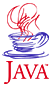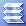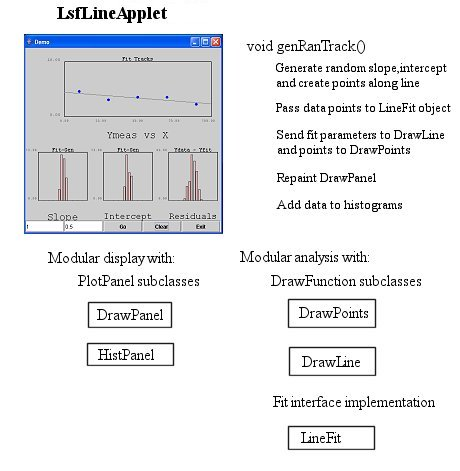Home : Map : Chapter 8 : Java : Tech : Physics : Least Squares Fit to a Straight LineIntroduction Threads Overview   Demo 1   Demo 2 Stopping Threads Multi-Processing Thread Tasks Animations   Demo 3   Demo 4     Demo 5 Non-interacting   Demo 6 Task Splitting   Demo 7 Exclusivity   Demo 8 Communicating   Demo 9 Priority/Scheduling More Thread Exercises
 About JavaTech      Codes List      Exercises      Feedback      References      Resources      Tips      Topic Index      Course Guide      What's New

Finding a function that fits a set of data is a common requirement in the analysis of an experiment. The Least Squares Fit (LSF) method leads to a set of equations that provide estimates of the parameters of a function, such as the intercept and slope of a straight line, to fit to a set of data. Furthermore, it provides error estimates for these parameters.

The LSF begins with the definition of a chi-square function as in .

chi2 = sumNi=1[ (yi - f(xi, a1,a2,...aM)/ sigmai]2

for the case of a N values of a single independent variable y and a single dependent variable x. (See, for example Press, for a discussion of the derivation of the chi-square function from maximum likelihood estimation.) Here yi is to the measured value at dependent variable point xi and f(xi, a1,a2,...aM) is a function of x with M parameters. The error on the yi measurement is give by sigma. which corresponds to the standard devivation value on the distribution of yi measurements.

Minimizing the chi-square with respect to the parameters, as in

d(chi2)/daj = 0, for j=1,..,M

will lead to a set of equations that can be solved for the parameters. We will not derive the solutions for particular functions here. See the references below or other numerical analysis books for details.

For a straight line and errors sigmai;

y = a + bx

results in (ref. Press)

a = (SxxSy - SxSxy)/D
b = (SSxy - SxSy)/D

sigmaa2 = Sxx/D
sigmab2 = S/D

where

S   = sumNi=1[1/sigmai2]
Sx  = sumNi=1[xi/sigmai2]
Sy  = sumNi=1[yi/sigmai2]
Sxy = sumNi=1[xi*yi/sigmai2]
Sxx = sumNi=1[xi*xi/sigmai2]

D = SSxx - (Sx)2

The following applet generates "tracks" (lines between left and right sides) and then selects points along the horzontal axis to make vertical measurements of the tracks tracks. The user can select a smearing factor (std.dev. of Gaussian) for the vertical measurements. The program fits a track to the points using the above formulas and plots it. Differences in the generated and fitted slope and intercept values are displayed in two histograms. The third histogram shows the distribution of the residuals (differences between the fitted track values and the measured values).

This program uses a highly modular approach that brings a lot flexibility. We will use the classes here in many progams in following chapters. The following diagram highlights the main elements of the LsfLine_JApplet11 program:The method genRanTrack() in class LsfLine_JApplet11 creates a random track and finds points along the track to simulate measured values. It then fits these points using an instance of the LineFit class. The fit parameters and the points go to instances of the DrawLine and DrawPoints classes, respectively. These two are both subclasses of DrawFunction. The DrawPanel loops through its array of DrawFunction objects and invokes the draw() method of each.

LsfLineApplet.java - This program generates points along a line and then fits a straight line to them. This simulates track fitting in a detector. The number of tracks and the std. dev. of the smearing of the track measurement errors taken from entries in two text fields. Two histograms record differences in the slope and intercept for the fitted track with the generated track. A third histogram holds the residuals.

+ New classes:
FitLine.java - least squares fit to a straight line.
Fit.java - abstract class that allows for different kinds of data fitting algorithms to 2-D data by subclasses.

+ Previous classes:
Chapter 6:Tech: DrawFunction.java, DrawLine.java, DrawPoints.java, DrawPanel.java
Chapter 6:Tech: Histogram.java, HistPanel.java
Chapter 6:Tech: PlotPanel.java, PlotFormat.java

Java Notes

• Modularization and polymorphism are illustrated again here by the use of the DrawFunction objects and the Fit base class and its subclass FitLine. The program uses objects for all the primary activities and allows for the classes to be re-uised in other programs.

import javax.swing.*;
import java.awt.*;
import java.awt.event.*;

/**
*
*  It generates points along a line and then fits a
*  straight line to them. This simulates track fitting
*  in a detector.
*
*  The number of tracks and the SD of
*  the smearing of the track measurement errors taken from
*  entris in two text fields. Two histograms record differences
*  in the slope and intercept for the fitted track with the
*  generated track. A third histogram holds the residuals.
*
*  This program will run as an applet inside
*  an application frame.
*
*  The "Go" button starts the track generation and fitting in a
*  thread. "Clear"  button clears the histograms.
*  In standalone mode, the Exit button closes the program.
*
**/
public class LsfLineApplet extends JApplet
implements ActionListener, Runnable
{
// Use the HistPanel JPanel subclass here
HistPanel fSlopePanel;
HistPanel fInterceptPanel;
HistPanel fResidualsPanel;

// Use a DrawPanel to display the points to fit
DrawPanel fDrawPanel = null;

// Thee histograms to record differences between
// generated tracks and fitted tracks.
Histogram fSlopeHist;
Histogram fInterceptHist;
Histogram fResidualsHist;

// Use DrawFunction subclasses to plot on the DrawPanel
DrawFunction [] fDrawFunctions;

// Set values for the tracks including the default number
// of tracks to generate, the track area, the SD smearing
// of the data points, and the x values where the track
// y coordinates are measured.
int fNumTracks   =     1;
double fYTrkMin  =   0.0;
double fYTrkMax  =  10.0;
double fXTrkMin  =   0.0;
double fXTrkMax  = 100.0;

double fTrkSmear = 0.5;

double [] fXTrk  = {10.0,30.0,50.0,70.0,90.0};
double [] fYTrk  = new double;

// Data array used to pass track points to DrawPoints
double [][] fData = new double[];

// Random number generator
java.util.Random fRan;

// Inputs for the number of tracks to generate
JTextField fNumTrksField;
// and the smearing of the tracking points.
JTextField fSmearField;

// Flag for whether the applet is in a browser
// or running via the main () below.
boolean fInBrowser = true;

//Buttons
JButton fGoButton;
JButton fClearButton;
JButton fExitButton;

// Use thread reference as flag.

/**
* Create a User Interface with histograms and buttons to
* control the program. Two text files hold number of tracks
* to be generated and the measurement smearing.
**/
public void init () {

// Will need random number generator for generating tracks
// and for smearing the measurement points
fRan = new java.util.Random ();

// Create instances of DrawFunction for use in DrawPanel
// to plot the tracks and the measured points along them.
fDrawFunctions    = new DrawFunction;
fDrawFunctions = new DrawLine ();
fDrawFunctions = new DrawPoints ();

// Start building the GUI.
JPanel panel = new JPanel (new GridLayout (2,1));

// Will plot the tracks on an instance of DrawPanel.
fDrawPanel =
new DrawPanel (fYTrkMin,fYTrkMax, fXTrkMin, fXTrkMax,
fDrawFunctions);

fDrawPanel.setTitle ("Fit Tracks");
fDrawPanel.setXLabel ("Ymeas vs X");

// Create histograms to show the quality of the fits.
fSlopeHist  = new Histogram ("Fit-Gen","Slope", 20, -0.1,0.1);
fInterceptHist = new Histogram ("Fit-Gen","Intercept", 20, -5.,5.);
fResidualsHist  = new Histogram ("Ydata - Yfit","Residuals", 20, -3,3.);

// Use another panel to hold the histogram and controls panels.
JPanel hist_crls_panel = new JPanel (new BorderLayout ());

JPanel histsPanel = new JPanel (new GridLayout (1,3));

// Add the panel of histograms to the main panel

// Use a textfield for an input parameter.
fNumTrksField =
new JTextField (Integer.toString (fNumTracks), 10);

// Use a textfield for an input parameter.
fSmearField =
new JTextField (Double.toString (fTrkSmear), 10);

// If return hit after entering text, the
// actionPerformed will be invoked.

fGoButton = new JButton ("Go");

fClearButton = new JButton ("Clear");

fExitButton = new JButton ("Exit");

JPanel control_panel = new JPanel (new GridLayout (1,5));

if (fInBrowser) fExitButton.setEnabled (false);

// Add text area with scrolling to the applet.

} // init

public void actionPerformed (ActionEvent e)  {
Object source = e.getSource ();
if ( source == fGoButton || source == fNumTrksField
|| source == fSmearField  )
{
String strNumDataPoints = fNumTrksField.getText ();
String strTrkSmear = fSmearField.getText ();
try {
fNumTracks = Integer.parseInt (strNumDataPoints);
fTrkSmear = Double.parseDouble (strTrkSmear);
}
catch (NumberFormatException ex) {
// Could open an error dialog here but just
// display a message on the browser status line.
return;
}

fGoButton.setEnabled (false);
fClearButton.setEnabled (false);
if (fThread != null) stop ();

}
else if (source == fClearButton) {
fSlopeHist.clear ();
fInterceptHist.clear ();
fResidualsHist.clear ();

repaint ();
}
else if (!fInBrowser)
System.exit (0);

} // actionPerformed

/** Stop thread by setting reference to null. This stops
* loop in run()
**/
public void stop (){
// If thread is still running, setting this
// flag will kill it.
} // stop

/** Generate the tracks in a thread. */
public void run (){

for (int i=0; i < fNumTracks; i++){
// Stop the thread if flag set

// Generate a random track.
double [] genParams = genRanTrack (fXTrkMax-fXTrkMin,
fYTrkMax-fYTrkMin,
fXTrk, fYTrk, fTrkSmear);

// Fit points to a straight line. Use constant error.
double [] fitParams = new double;
FitLine.fit (fitParams, fXTrk, fYTrk, null, null, fXTrk.length);

// Pass the parameters to the straight line fit.
fDrawFunctions.setParameters (fitParams,null);

// Pass the data points to the DrawPoints object via
// the 2-D array.
fDrawFunctions.setParameters (null, fData);

// Redrawing the panel will cause the paintContents (Graphics g)
// method in DrawPanel to invoke the draw () method for the line
// and points drawing functions.
fDrawPanel.repaint ();

// Add fit measurement values to the respective histograms.

// Include residuals == difference between the measured value
// and the fitted value at the points at each x position
for (int j=0; j < fXTrk.length; j++){
double y = fitParams + fitParams*fXTrk[j];
}

// Pause briefly to let users see the track.
try
{
}
catch (InterruptedException e){}
}

repaint ();

fGoButton.setEnabled (true);
fClearButton.setEnabled (true);
} // run

/**
*  Generate a track and obtain points along the track.
*  Smear the vertical coordinate with a Gaussian.
**/
double [] genRanTrack (double xRange, double yRange,
double [] xTrack,double [] yTrack,
double smear) {

// Line intercept and slope values in array.
double [] lineParam = new double;

// Simulated tracks for creating the desired distibution.
double y0 = yRange * fRan.nextDouble ();
double y1 = yRange * fRan.nextDouble ();

lineParam =  (y1-y0)/xRange;

for (int i=0; i < xTrack.length; i++){
yTrack[i] = y0 + lineParam*xTrack[i];
yTrack[i] += smear*fRan.nextGaussian ();
}

// Set up the parameters in the drawing function.
lineParam = y0; //intercept
fDrawFunctions.setParameters (lineParam,null);

// The LineFit function will need this data via
// a 2-D array.
fData = yTrack;
fData = xTrack;
fData = null;
fData = null;

// Return the track parameters.
return lineParam;

} // genRanTrack

/**
*  Allow for option of running the program in standalone mode.
*  Create the applet and add to a frame.
**/
public static void main (String[] args) {
//
int frame_width=450;
int frame_height=450;

//
LsfLineApplet applet = new LsfLineApplet ();
applet.fInBrowser = false;
applet.init ();

// Following anonymous class used to close window & exit program
JFrame f = new JFrame ("Demo");
f.setDefaultCloseOperation (JFrame.EXIT_ON_CLOSE);

// Add applet to the frame
f.setSize (new Dimension (frame_width,frame_height));
f.setVisible (true);
} // main

} // LsfLineApplet

import java.awt.*;

/**
*  Fit straight line to a set of data points.
*  Implements the Fit interface.
*
**/
public class FitLine extends Fit
{

/**
*  Use the Least Squares fit method for fitting a
*  straight line to 2-D data for measurements
*  y[i] vs. dependent variable x[i]. This fit assumes
*  there are errors only on the y measuresments as
*  given by the sigma_y array.

*  See, e.g. Press et al., "Numerical Recipes..." for details
*  of the algorithm.
**/
public static void fit (double [] parameters, double [] x, double [] y,
double [] sigma_x, double [] sigma_y, int num_points){

double s=0.0,sx=0.0,sy=0.0,sxx=0.0,sxy=0.0, del;

// Null sigma_y implies a constant error which drops
// out of the divisions of the sums.
if (sigma_y != null){
for (int i=0; i < num_points; i++){

s   += 1.0/ (sigma_y[i]*sigma_y[i]);
sx  += x[i]/ (sigma_y[i]*sigma_y[i]);
sy  += y[i]/ (sigma_y[i]*sigma_y[i]);
sxx +=  (x[i]*x[i])/ (sigma_y[i]*sigma_y[i]);
sxy +=  (x[i]*y[i])/ (sigma_y[i]*sigma_y[i]);
}
} else {
s = x.length;
for (int i=0; i < num_points; i++){
sx  += x[i];
sy  += y[i];
sxx += x[i]*x[i];
sxy += x[i]*y[i];
}
}

del = s*sxx - sx*sx;

// Intercept
parameters =  (sxx*sy -sx*sxy)/del;
// Slope
parameters =  (s*sxy -sx*sy)/del;

// Errors  (sd**2) on the:
// intercept
parameters = sxx/del;
// and slope
parameters = s/del;

} // fit

} // FitLine

import java.awt.*;

/** Interface for algorithms to fit 2-D data points. */
public class Fit
{
/**
*  Returns an array with the fit parameters, e.g.
*  slope and intercept for a straight line, to 2-D
*  data.
**/
public static void fit (double [] parameters, double [] x, double [] y,
double [] sigma_x, double [] sigma_y, int num_points) {};

} // Fit

References & Web Resources

Last update: Feb.27.04

 Tech Timers   Demo 1 Hist. Adapt Range   Demo 2 Sorting in Java   Demo 3 Histogram Median   Demo 4 Refactoring   Demo 5 Error Bars   Demo 6 Exercises
 Physics Least Squares Fit   Demo 1 Fit to Polynomial   Demo 2 Fit Hist Errors   Demo 3 Discretization   Demo 4 Timing   Demo 5 ExercisesPart I Part II Part III Java Core 1  2  3  4  5  6  7  8  9  10  11  12 13 14 15 16 17 18 19 20 21 22 23 24 Supplements 1  2  3  4  5  6  7  8  9  10  11  12 Tech 1  2  3  4  5  6  7  8  9  10  11  12 Physics 1  2  3  4  5  6  7  8  9  10  11  12

Java is a trademark of Sun Microsystems, Inc.